## RS Aggarwal Solutions Class 10 Chapter 18 Areas of Circle, Sector and Segment Ex 18a

These Solutions are part of RS Aggarwal Solutions Class 10. Here we have given RS Aggarwal Solutions Class 10 Chapter 18 Areas of Circle, Sector and Segment Ex 18a.

Other Exercises

Question 1.
Solution: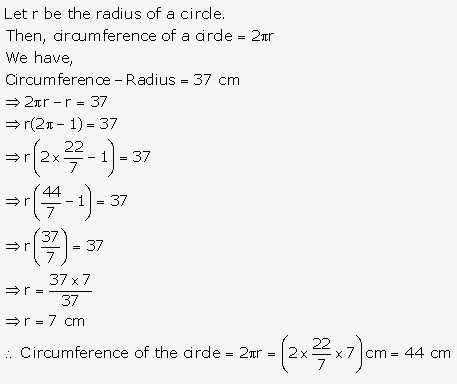Question 2.
Solution: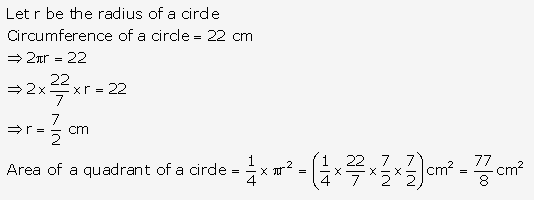Question 3.
Solution:Question 4.
Solution: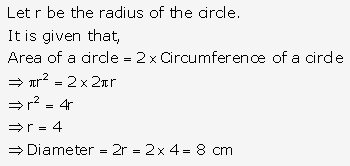Question 5.
Solution:
Since square circumscribes a circle of radius a cm, we have
Side of the square = 2 ⨯ radius of circle = 2a cm
Then, Perimeter of the square = (4 ⨯ 2a) = 8a cm

Question 6.
Solution: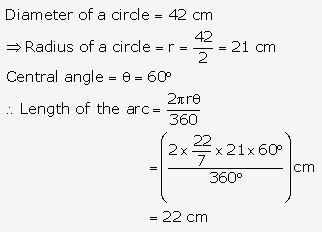Question 7.
Solution: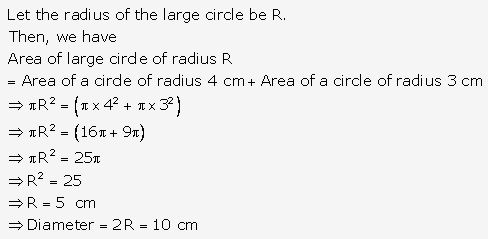Question 8.
Solution:Question 9.
Solution:Question 10.
Solution:Question 11.
Solution: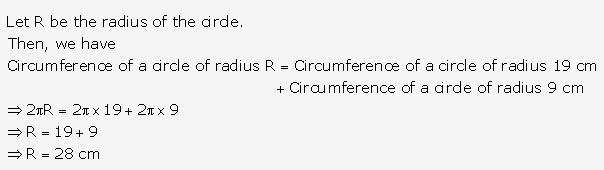Question 12.
Solution: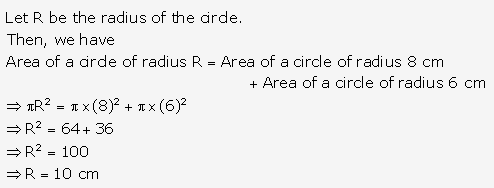Question 13.
Solution: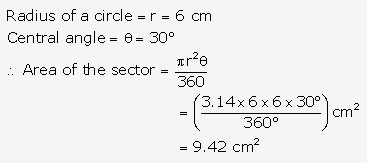Question 14.
Solution: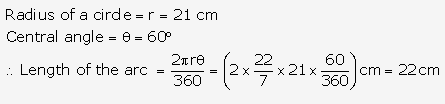Question 15.
Solution: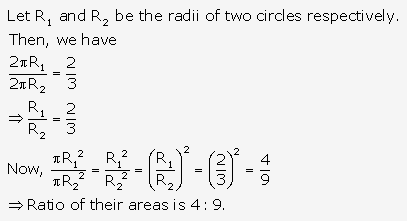Question 16.
Solution: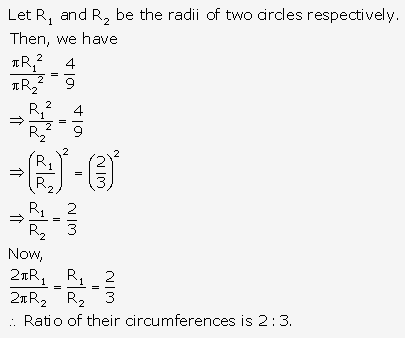Question 17.
Solution:Question 18.
Solution:Question 19.
Solution:
Length of the pendulum = radius of sector = r cmQuestion 20.
Solution:
Angle described by the minute hand in 60 minutes = 360°
Angle described by minute hand in 20 minutes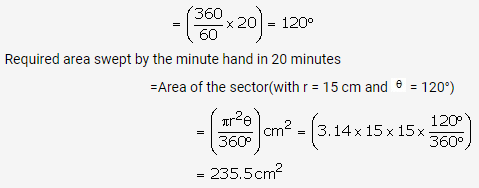Question 21.
Solution:Question 22.
Solution: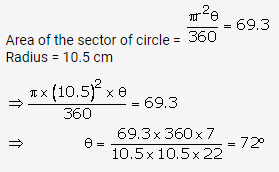Question 23.
Solution:
Let sector of circle is OAB
Perimeter of a sector of circle = 31 cm
OA + OB + length of arc AB = 31 cm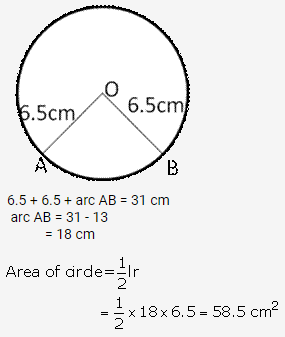Question 24.
Solution:
Length of arc of circle = 44 cm
Radius of circle = 17.5 cm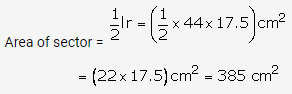Question 25.
Solution: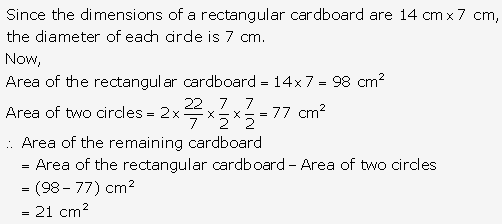Question 26.
Solution: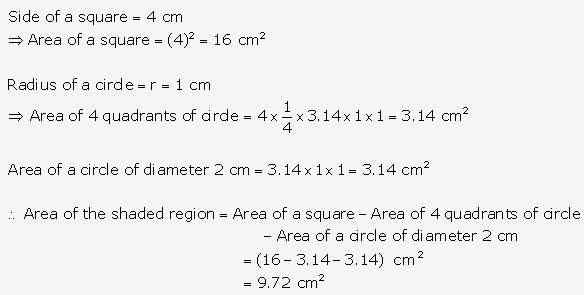Question 27.
Solution: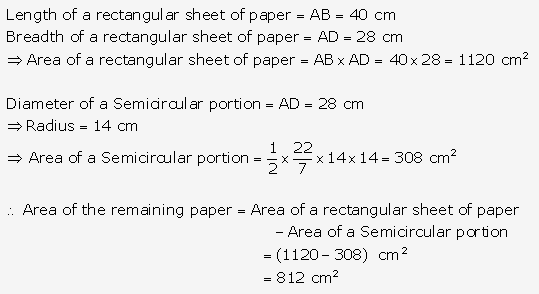Question 28.
Solution: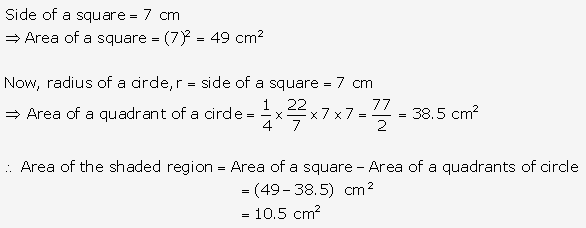Question 29.
Solution: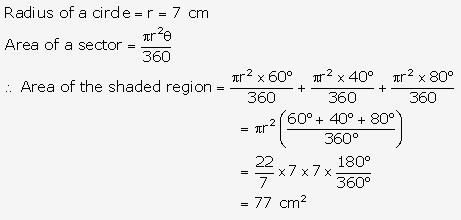Question 30.
Solution: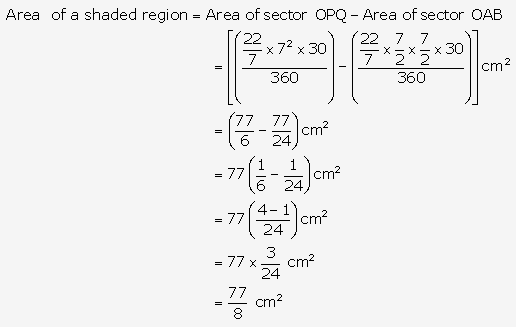Question 31.
Solution: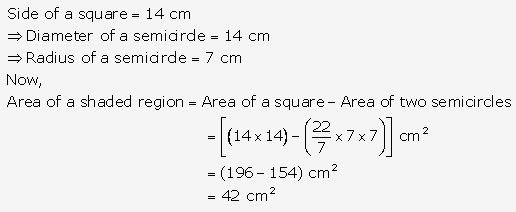Question 32.
Solution: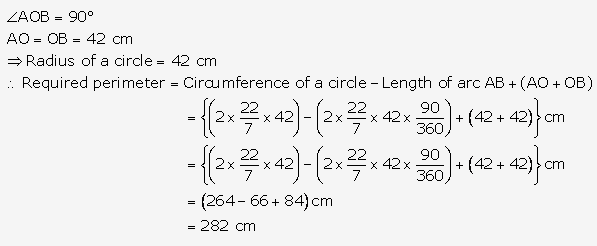Question 33.
Solution: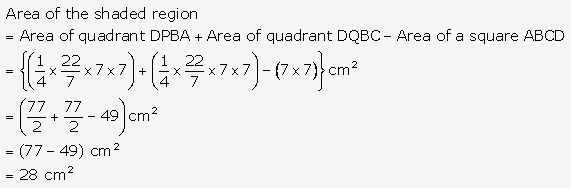Question 34.
Solution:Question 35.
Solution: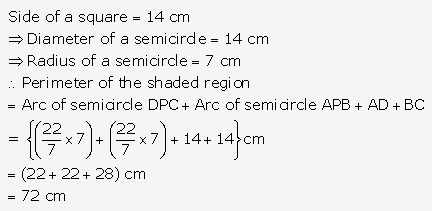Question 36.
Solution: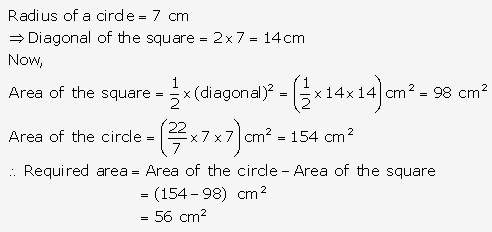Question 37.
Solution:Question 38.
Solution: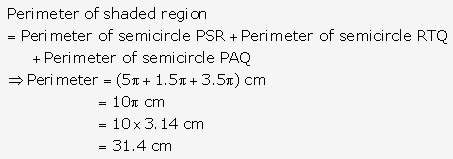Question 39.
Solution:Question 40.
Solution: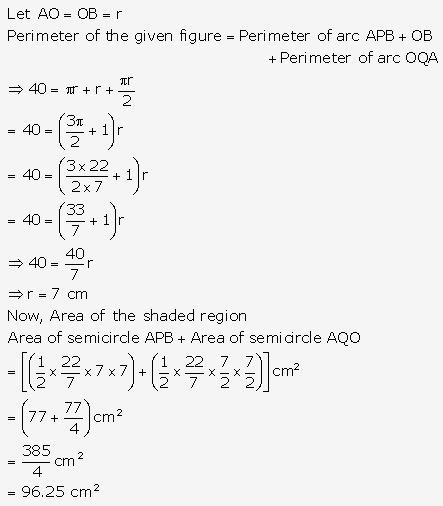Question 41.
Solution: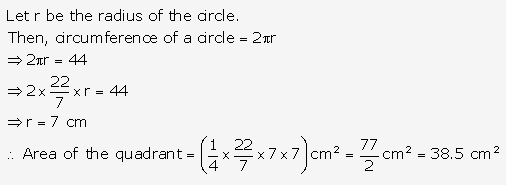Question 42.
Solution: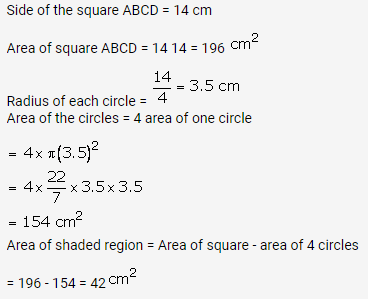Question 43.
Solution: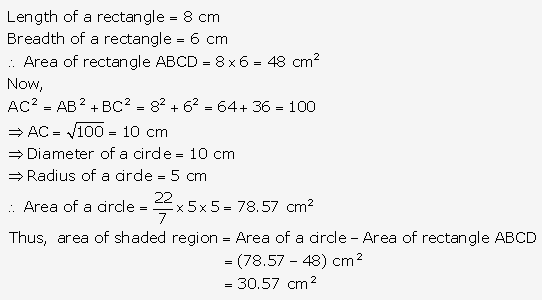Question 44.
Solution: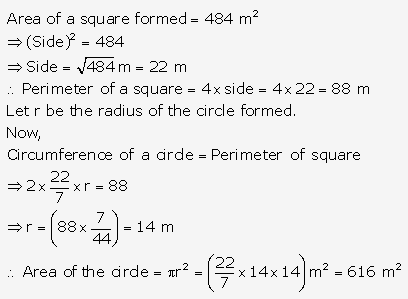Question 45.
Solution: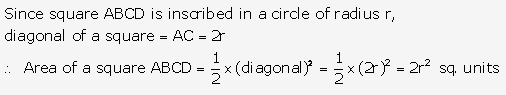Question 46.
Solution:Question 47.
Solution:Question 48.
Solution:
Area of flower bed = (area of quadrant OPQ) – (area of the quadrant ORS)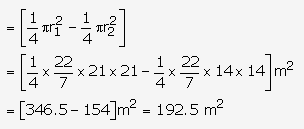Question 49.
Solution: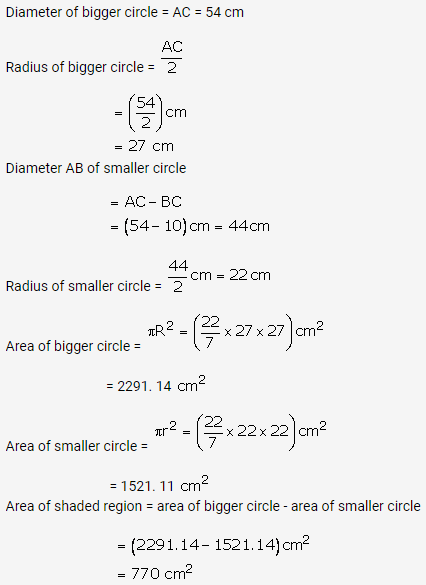Question 50.
Solution:Question 51.
Solution: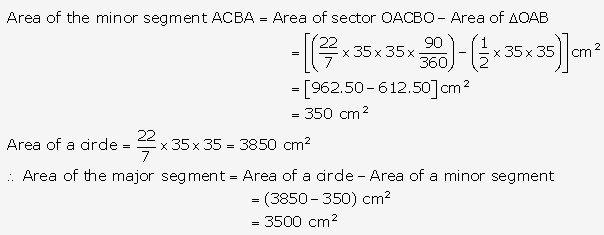Hope given RS Aggarwal Solutions Class 10 Chapter 18 Areas of Circle, Sector and Segment Ex 18a are helpful to complete your math homework.

If you have any doubts, please comment below. Learn Insta try to provide online math tutoring for you.